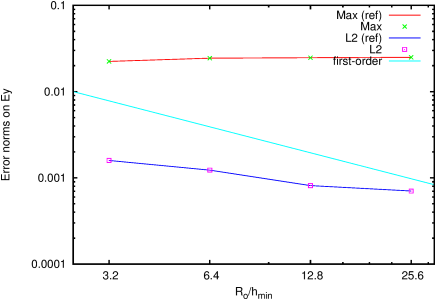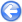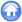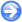### 14.3PASS: Charge relaxation in an axisymmetric insulated conducting column

Author
J.M. López-Herrera and S. Popinet
Command
sh cylinder.sh cylinder.gfs
Version
100609
Required files
cylinder.sh norms.ref
Running time
5 seconds

A conducting rigid cylinder of radius Ro=0.1 is immersed in an insulating medium. Initially an uniform charge volume density is set in the cylinder (ρe (x,0)=0.5). As time passes the charge migrates from the bulk to the interface of the cylinder but the total charge in the cylinder section is preserved.

Q(t)=
 Σ
ρe(x,t)   d Σ = Qo= π Ro2   ρe(x,0).

If a VariableVOFConcentration is used instead of a VariableTracer the total charge is also preserved. The outer electric field reaches a steady-state (Figure 204).

A more detailed discussion of this simulation is given in .

 Figure 203: Relative error on the charge per unit of length of cylinder as a function of resolution. (% Error =|1−Q(t)/Qo)|)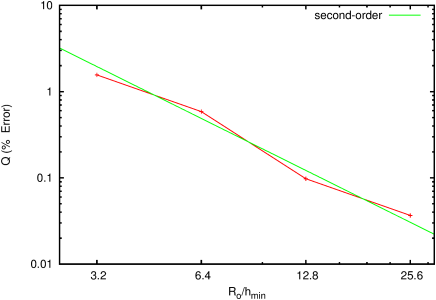Figure 204: Steady-state electric field profile as a function of resolution.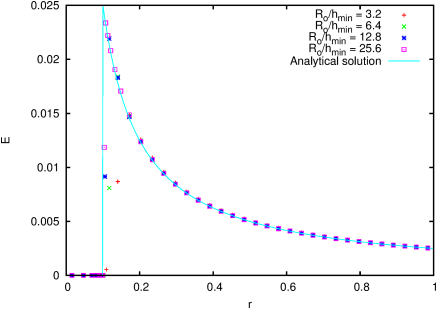Figure 205: Error norms for the electric field.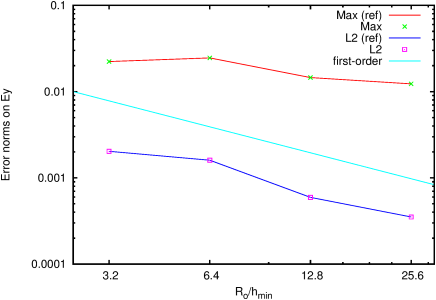#### 14.3.1PASS: Charge relaxation in a planar cross-section

Author
J.M. López-Herrera and S. Popinet
Command
sh ../cylinder.sh planar.gfs
Version
100609
Required files
norms.ref
Running time
10 seconds

Same problem but solved in a planar cross-section of the column.

 Figure 206: Relative error on the charge per unit of length of cylinder as a function of resolution. (% Error =|1−Q(t)/Qo)|)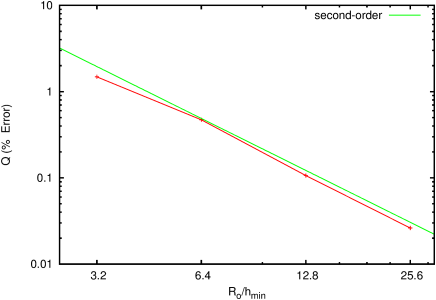Figure 207: Steady-state electric field profile as a function of resolution.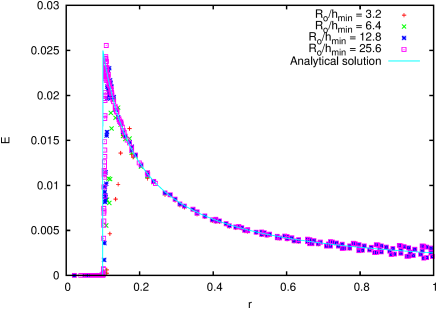Figure 208: Error norms for the electric field.